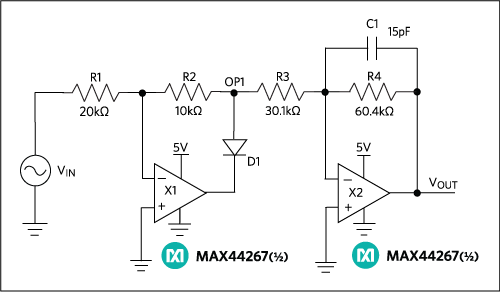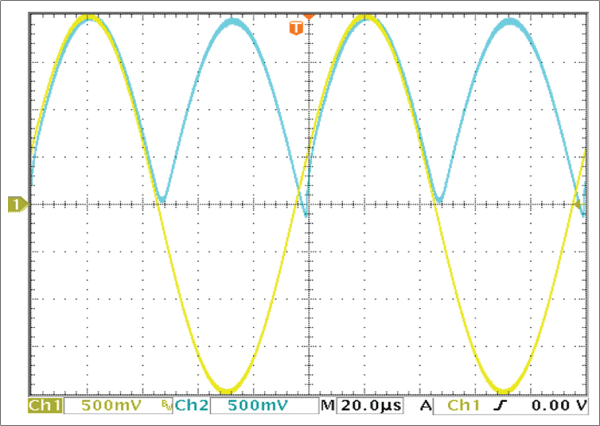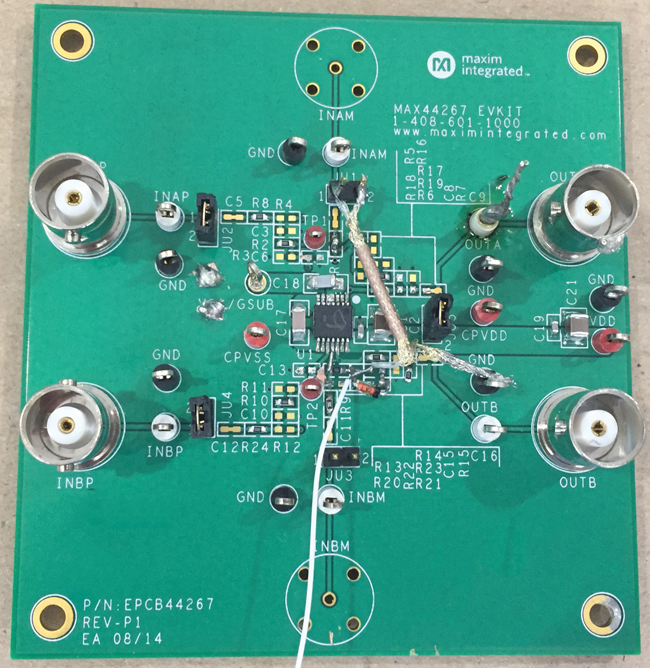# Build a Full-Wave Rectifier Circuit with a Single-Supply Op Amp

### 要約

How to build a full-wave rectifier of a bipolar input signal using the MAX44267 single-supply, dual op amp.

### Introduction

Implementing simple functions in a bipolar signal environment when working with single-supply op amps can be quite a challenge because, oftentimes, additional op amps and/or other electronic components are required. The alternative is the MAX44267, which has a unique built-in charge pump that permits split-rail performance with only a single supply.

### Circuit Design

The circuit in Figure 1 uses the MAX44267 single-supply, dual op amp with a true-zero output to implement a full-wave rectifier with only a single supply rail. This circuit has been around for quite a long time. It requires a negative supply so the X1 amplifier can output a negative voltage of -0.5 times the input voltage. Note that when the input is positive, X1 has a gain of -0.5V/V plus a diode drop so that the OP1 node is exactly -0.5x the input.

R1, R2, and R3 are standard values, while R4 is easily implemented with two 120kΩ resistors in parallel. The ratios of all four resistors are important: R2 = 0.5 × R1; R4 = 2 × R3; and R1 + R2 + R3 = R4. Diode D1 can be any low-leakage signal diode such as 1N914. Capacitor C1 helps to reduce the MAX44267’s charge-pump noise.

When the input swings negative, X1 is cut off by diode D1, and the OP1 node is again at half the input voltage due to the resistive division, R1 + R2 to R3. Amplifier X2 then provides a further gain of -2V/V to correct the earlier 50% attenuation.At low frequency the output is almost error free. At the output’s zero crossings in Figure 2, there is barely 8mV of distortion (blue trace). This is caused because the X1 amplifier has to recover from being cut off by D1. Unlike most amplifiers with only a single supply, however, the output does reach a true zero output when the input passes through zero.As the frequency increases larger distortions start to appear at the output. Below are scope shots showing a variety of input amplitudes and frequencies. Figures 3, 4 and 5 show a 200mVP-P input signal at 200Hz, 1kHz, and 10kHz, respectively.This data illustrate the frequency limitation of this circuit topology. Specifically, op amp X1 takes a finite time to recover from being open loop and having to slew at its maximum rate to catch up with the input.

So far, only small signals have been shown, but this topology also handles larger signal amplitudes. Note that while the waveforms look much better, the trace scaling hides the errors that were visible on the low-amplitude signals.

Figures 6, 7 and 8 show a 4VP-P input signal at 200Hz, 1kHz, and 10kHz, respectively.### Summary

Within the limitations of the amplifier’s bandwidth, slew rate, and settling performance, a wide range of signal amplitudes and frequencies can be rectified with little error. The simple ratios for the four resistor values allow trim-free assembly, while the chopper-stabilized amplifier keeps both offset and drift to negligible levels.

Typically, full-wave rectification of a bipolar input signal requires circuitry running from split supplies. Yet, most systems run from a single supply like the solution shown here.